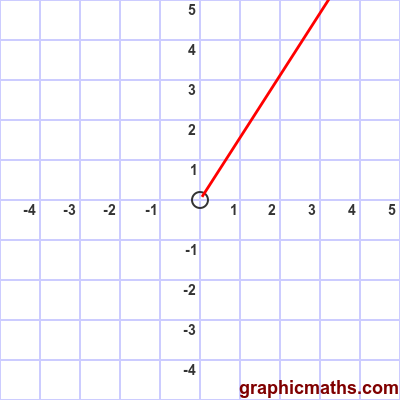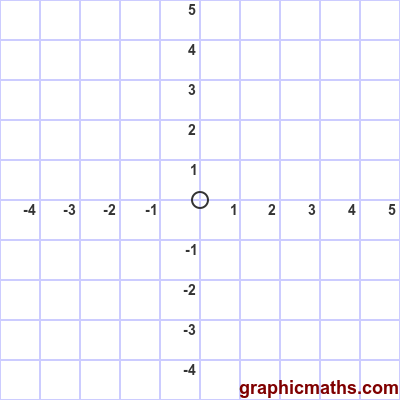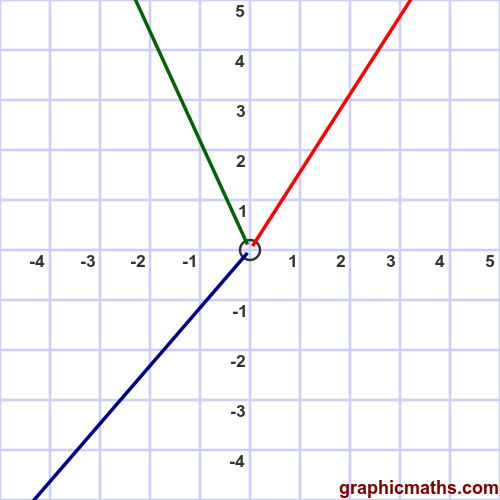# Polar curve constant theta

By Martin McBride, 2020-09-18
Tags: standard curves
Categories: polar coordinatesHere is a polar function of constant $\theta$.

$$\theta = 1$$

This function describes a curve where $\theta$ is constant for any value of $r$. In this case the angle is always 1 radian. The curve is the locus all points that are any distance $r$ from the origin in the direction of $\theta$. The curve in a half line, like this:Notice that the curve is a half line, because in polar coordinates, $r$ cannot be negative.

This animation shows how the curve is plotted as $r$ increases from 0:## Varying the angle

In general the function:

$$\theta = a$$

where $a$ is a constant between 0 and $2\pi$, describes a half line at an angle $a$, starring at the origin.

Here are the lines created for $n = 1$ (red), $n = 2$ (green), and $n = 4$ (blue):## Cartesian equation

We can convert the polar equation into a Cartesian equation using the identity:

$$\tan{\theta} = \frac {y}{x}$$

Rearranging this equation gives:

$$y = x \tan{\theta}$$

which is the Cartesian equation for a straight line of gradient $\tan \theta$, passing through the origin. However, the equation in only valid for the quadrant in which $r$ would be positive.